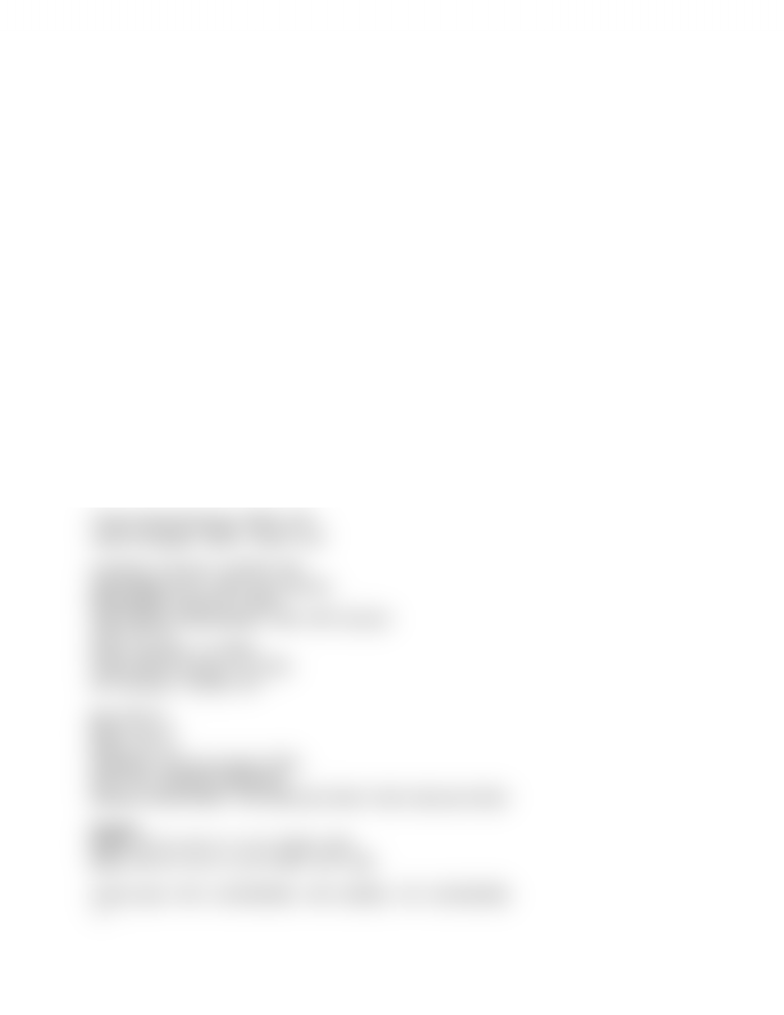# BUS 437 Study Guide - Final Guide: Shares Outstanding, Reserve Requirement

94 views2 pages
School
Course
Professor

For unlimited access to Study Guides, a Grade+ subscription is required.NWC = CA - CL
CFA = OCF - CS - Addition to NWC
OCF = EBIT + DEP - Taxes
CS = Ending FA - Beginning FA + DEP
Change in NWC = NWC end - NWC beg = (CA-CL)end - (CA-CL)beg
CF to creditors = INT - Net New LTD
CF to shareholders = Div - Net New Equity
CFA = CF to creditors + CF to shareholders
UCC(t) - CCA = UCC(t+1)
Salvage = MV - tax(MV-BV)
---
Current Ratio = CA / CL
Quick Ratio = (CA - Inv) / CL
Cash Ratio = Cash / CL
NWC to Total Assets = NWC / A
Interval Measure = CA / avg daily operating costs = CA / (COGS + INT)
TDR = (A-E)/A = D/A = 1-(E/A)
A = E+D
Debt/Equity = D/E
EM = A/E = 1 + D/E
Long Term Debt Ratio = LTD / (LTD + E)
Times Interest Earned = EBIT / INT
Cash Coverage = (EBIT + DEP) / INT
Inventory Turnover = COGS / INV
Days Sales in Inv = 365 / Inv Turnover
Receivables Turnover = S/AR
Days Sales in Receivables = 365 / AR Turnover
TAT = S / TA
NWC Turnover = S / NWC
Fixed Asset Turnover = S / NFA
AP Turnover = COGS / AP
pm = NI / S
ROA = NI / A
ROE = NI / E
PE Ratio = Price per share / EPS
EPS = NI / Shares outstanding
Market to Book Ratio = mkt value per share / book value per share
Dupont
ROE = NI / E = NI / A * A / E = ROA * EM
ROE = NI / S * S / A * A / E = PM * TAT * EM
Cash Cycle = INV / (COGS/365) + AR / (S/365) - AP / (COGS/365)
----
Unlock document

This preview shows half of the first page of the document.
Unlock all 2 pages and 3 million more documents.

Already have an account? Log in

# Get access

Grade+
\$10 USD/m
Billed \$120 USD annually
Homework Help
Class Notes
Textbook Notes
40 Verified Answers
Study Guides
1 Booster Class